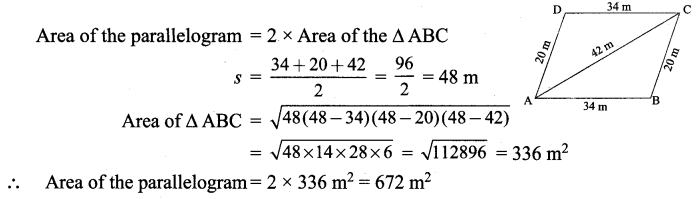## Tamilnadu Samacheer Kalvi 9th Maths Solutions Chapter 7 Mensuration Ex 7.1

9th Maths Mensuration Exercise 7.1 Question 1.
Using Heron’s formula, find the area of a triangle whose sides are
(i) 10 cm, 24 cm, 26 cm
(ii) 1.8 m, 8 m, 8.2 m
Solution:
(i) sides : 10 cm, 24 cm, 26 cm
Using Heron’s formula
Area of the triangle = $$\sqrt{s(s-a)(s-b)(s-c)}$$ sq. units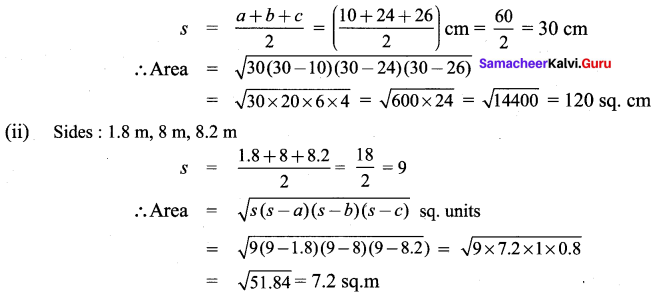9th Maths Mensuration Question 2.
The sides of the triangular ground are 22 m, 120 m and 122 m. Find the area and cost of leveling the ground at the rate of ₹ 20 per m2.
Solution: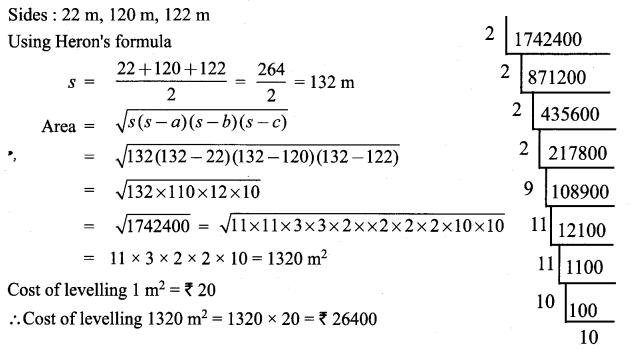9th Maths Book Mensuration ex 7.1 Question 3.
The perimeter of a triangular plot is 600 m. If the sides are in the ratio 5 : 12 : 13, then find the area of the plot.
Solution:
s = 600 m
Side s are in the ratio 5 : 12 : 13
5x + 12x + 13x = 30x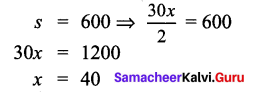∴ sides are 200 m, 480 m, 520 m.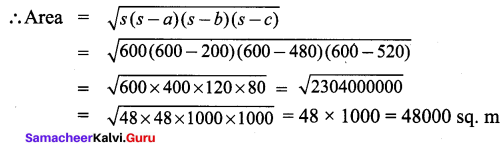9th Maths 7.1 Mensuration Question 4.
Find the area of an equilateral triangle whose perimeter is 180 cm.
Solution:
Perimeter of an equilateral triangle = 180 cm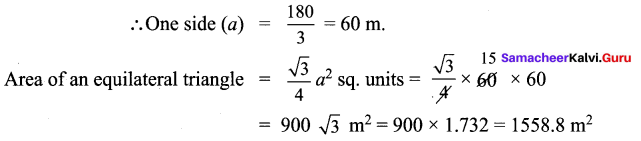9th Maths Exercise 7.1 Samacheer Kalvi Question 5.
An advertisement board is in the form of an isosceles triangle with perimeter 36m and each of the equal sides are 13 m. Find the cost painting it at ₹ 17.50 per square metre.
Solution: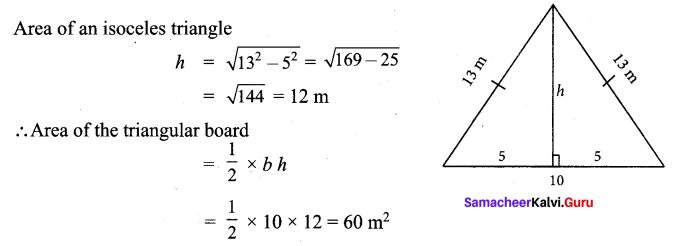Cost of painting 1 m2 = ₹ 17.50
Cost of painting 60m2 = 60 × 17.50 = ₹ 1050

9th Maths Mensuration Exercise 7.1 Question 6.
Find the area of the unshaded region.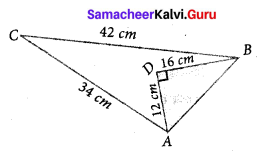Solution: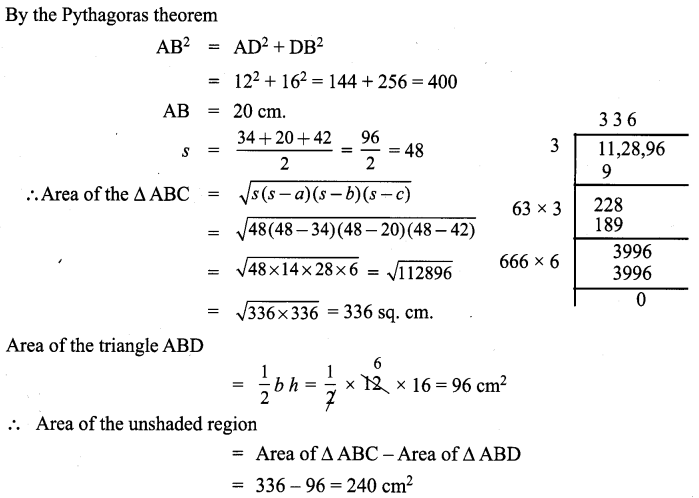10th Maths Exercise 7.1 Samacheer Kalvi Question 7.
Find the area of a quadrilateral ABCD whose sides are AB = 13 cm, BC = 12 cm, CD = 9 cm, AD = 14 cm and diagonal BD = 15 cm
Solution: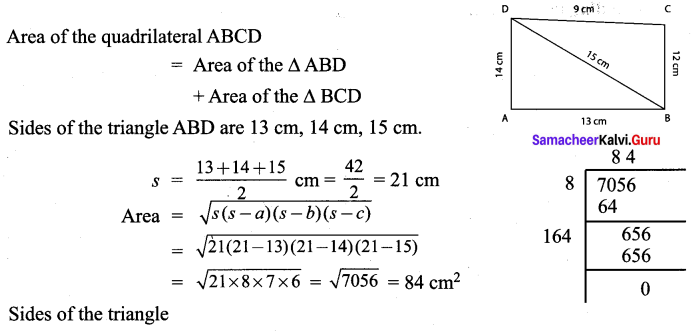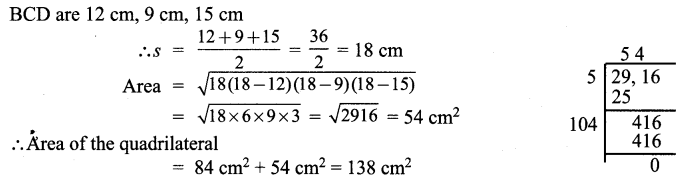9th Maths Exercise 7.1 Question 8.
A park is in the shape of a quadrilateral. The sides of the park are 15 m, 20m, 26 m and 17 m and the angle between the first two sides is a right angle. Find the area of the park.
Solution: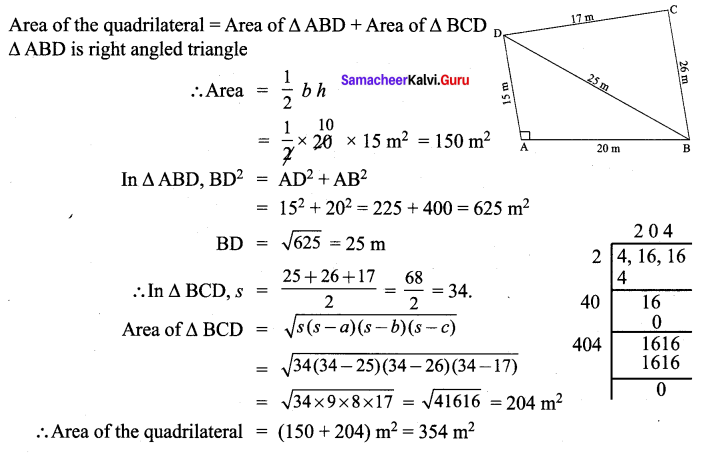Exercise 7.1 Class 10 Samacheer Kalvi Question 9.
A land is in the shape of rhombus. The perimeter of the land is 160 m and one of the diagonal is 48 m. Find the area of the land.
Solution:
Perimeter of the rhombus land = 160 m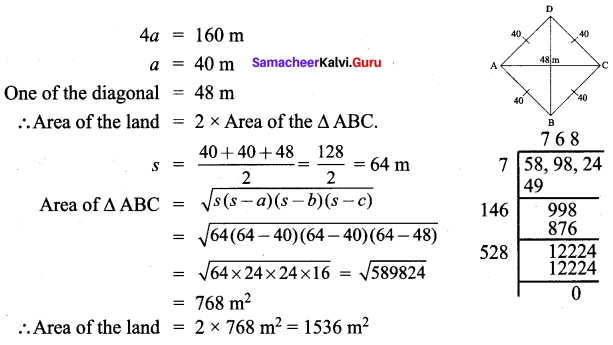9th Maths 7.1 Question 10.
The adjacent sides of a parallelogram measures 34 m, 20 m and the measure of the diagonal is 42 m. Find the area of Parallelogram.
Solution: